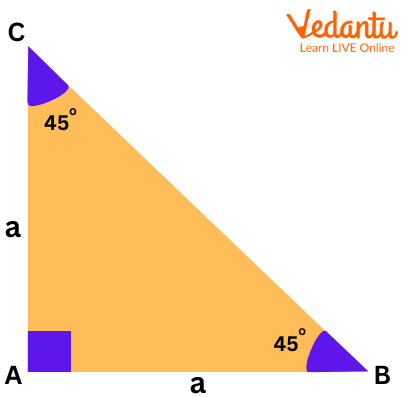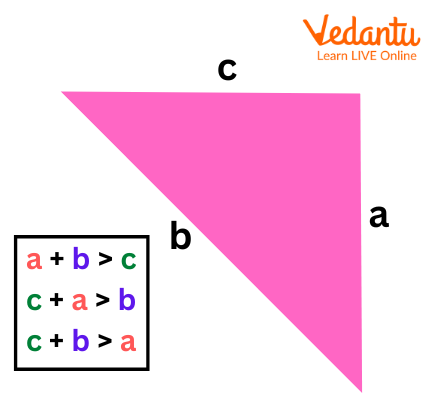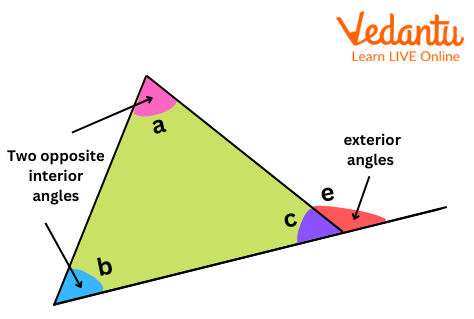Courses
Courses for Kids
Free study material
Free LIVE classes
More

# Properties of Triangle FormulaLIVE
Join Vedantu’s FREE Mastercalss

## Introduction:

Any three-sided polygon having three edges and three vertices is referred to as a triangle in geometry.

The fact that a triangle's interior angles add up to $180^\circ$ is its most crucial characteristic. Certain fundamental ideas, including the Pythagorean Theorem and trigonometry, depend on the characteristics of triangles.

## What are the Properties of a Triangle?

We must understand the many sorts of triangles in order to learn about the properties of triangles. Although all triangles have some characteristics, some of these characteristics depend on the sides and angles of the triangle.

## Angle Sum Property:

The angle sum property states that the sum of a triangle's three interior angles is always $180^\circ$.Angle Sum Property

In the given triangle, $\angle A + \angle B + \angle C = 180^\circ$

## Triangle Inequality Property

The length of a triangle's two sides added together is longer than its third side, according to the triangle inequality theorem.Inequality Property

## Pythagoras Theorem:

The hypotenuse square of a right-angled triangle is equal to the sum of the squares of the other two sides, according to the Pythagoras theorem. Mathematically, it is expressed as $Hypotenus{e^2} = Bas{e^2} + Altitud{e^2}$. See the altitude, base, and hypotenuse in the illustration below.

Side Opposite to the Greater Angle is the Longest Side:

Look at the triangle below to better grasp this triangle's property that the longest side is the one that is opposite the largest angle. B is the largest angle in this triangle. The side AC is hence the longest side.

## Exterior Angle Property:

The exterior angle of a triangle is always equal to the sum of the interior opposite angles, according to the outside angle theorem. Exterior angle (e) of the triangle presented is equal to $\angle a + \angle b$Exterior Angle Property

It should be remembered that a triangle can expand its three external angles, and the sum of all these exterior angles is $360^\circ$.

## Important Notes for Triangle:

• What is the formula of triangle area? The fundamental formula for determining a triangle's area is ${\rm{Area of triangle = }}\dfrac{1}{2} \times Base \times Height$.

• A triangle's perimeter is the sum of the lengths of its three sides.

## Conclusion:

To learn about the properties of triangles, we must first understand the many types of triangles. Although all triangles have some characteristics, some of these features are dependent on the triangle's sides and angles.

## Solved Example:

Example 1: A triangle has two angles that are $75^\circ$ and $60^\circ$ in length. Determine the third angle's measurement.

Solution: Two angles in a triangle have measurements of  an$75^\circ$and $60^\circ$.

Sum of two angles is $135^\circ = 75^\circ + 60^\circ$.

Using a triangle's characteristics, we can determine that the total of its three angles equals 180°.

So the third angle will be $180^\circ -135^\circ =45^\circ$.

Example 2: Tim is trying to build a triangle with sides that are 5 cm, 4 cm, and 9 cm long. Can he accomplish it?

Solution: The sides are 5 cm, 4 cm, and 9 cm long.

5 cm + 4 cm= 9 cm

In this case, the third side is equal to the total of the two smaller sides. However, according to the triangle inequality theorem, any two sides should add up to more than the third side.

Tim won't be able to build a triangle with sides of 5 cm, 4 cm, and 9 cm, according to the triangle's characteristics.

Example 3:  The sides of a triangle are given as 3 cm, 4 cm, and 5 cm. Calculate the perimeter of the triangle.

Solution: Sides of the triangle are: x = 3 cm, y = 4 cm and z = 5 cm

The perimeter of the triangle is given by

$\begin{array}{l}P = x + y + z\\P = 3 + 4 + 5\\P = 12cm\end{array}$

Therefore, the perimeter of the given triangle is 12 cm.

Last updated date: 28th Sep 2023
Total views: 68.1k
Views today: 1.68k

## FAQs on Properties of Triangle Formula

1. In maths, how many different kinds of triangles are there?

In general, there are six different kinds of triangles. They are right-angled triangles, acute triangles, obtuse triangles, scalene triangles, isosceles triangles, and equilateral triangles.

2. What is a Right-Angle Triangle?

A triangle that has one of the interior angles as $90^\circ$ is a right-angled triangle.

3. What is the Area of a Triangle?

The area of a triangle is equal to half of the product of its base and height. It is the space enclosed by the sides of the triangle and is expressed with the formula, ${\rm{Area of triangle = }}\dfrac{1}{2} \times Base \times Height$ . The area of a triangle is expressed in square units.

4. Describe the Angle Sum Property of a Triangle?

According to the Angle sum property of a triangle, the sum of the interior angles of a triangle is always$180^\circ$ . For example, if the 3 interior angles of a triangle are given as$\angle a,\angle b{\rm{ and }}\angle c$ , then this property can be expressed as, $\angle a + \angle b + \angle c = 180^\circ$

5. What are the Properties of a Right-angled Triangle?

The properties of a right-angled triangle are given as follows:

The largest angle is always $90^\circ$ which means it cannot have any obtuse angle.

The largest side is called the hypotenuse which is always the side opposite to the right angle.